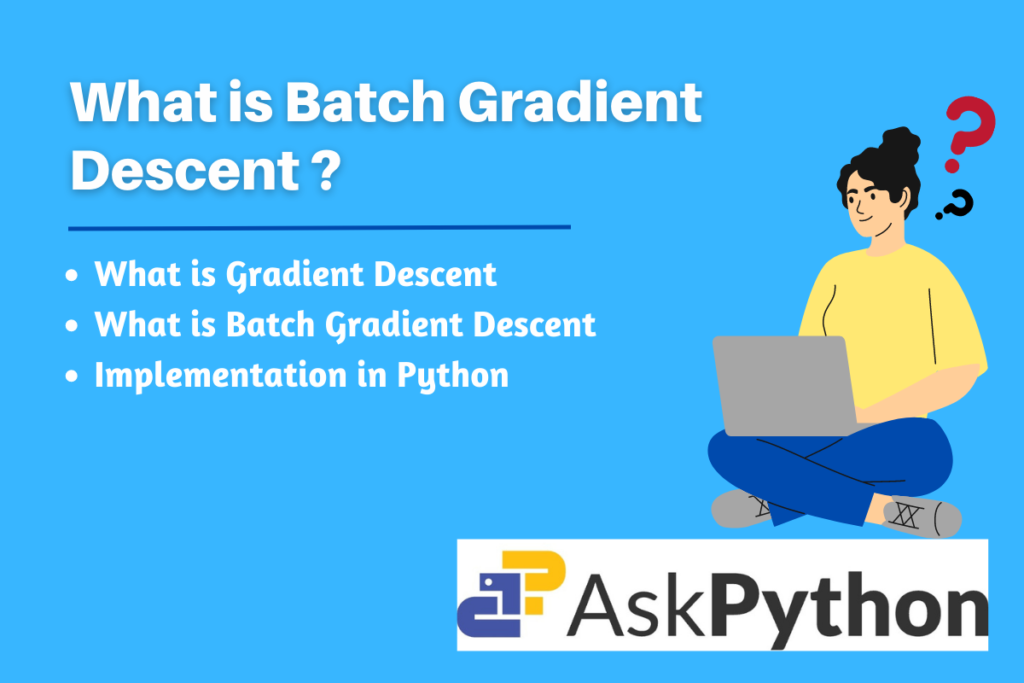# Mastering Batch Gradient Descent: A Comprehensive GuideDeep learning is in the lead when it comes to the most recent, widely employed technology. Let’s try to learn about the concept of batch gradient descent in machine learning in this post. It is among the most crucial subjects in deep learning, especially in supervised machine learning

Before getting started with Batch gradient descent, it is essential to understand what gradient descent is and why it is implemented.

The machine learning model makes an effort to “learn” from the supplied data. It uses data analysis to find patterns, with little to no human input. The machine learning model learns from its own faults that arise as a result, just like human beings learn from their mistakes. Gradient descent is an iterative optimization algorithm that is employed for this training purpose.

To put it another way, a gradient calculates how much a function’s output will change if its inputs are slightly altered. A gradient merely quantifies the change in all weights relative to the error change. A gradient can alternatively be thought of as a function’s slope. A model can learn more quickly when the gradient is greater since it causes the slope to be steeper.

To reduce a predefined loss function is the objective of gradient descent. It completes two main phases iteratively in order to accomplish this objective. First, determine the slope (gradient), which is the current point’s first-order derivative of the function. From the current location, move the calculated distance in the opposite direction of the slope up.

## What is Batch Gradient Descent?

In batch gradient descent, each step is determined by taking into account all the training data. The parameters are only changed once all training examples have been evaluated once, and the error is determined for each example in the training data set. Using the mean gradient, we update our parameters by averaging the gradients of all the training samples. Therefore, that is only one gradient descent step in one epoch.

## Implementing a Simple Batch Gradient Descent Algorithm in Python

The algorithm is quite straightforward to use. Before predicting the output (y_pred), we initialize the weights (w) to zero. Then, we multiply the weights by the specified input training examples (X * W).

After we have the predicted output, we can calculate the derivative of the loss function with respect to W using the predicted output (y_pred) and the actual output. The derivative of the loss function with respect to W (dW) is calculated using the following formula

dW = XT . (y_p_pred – y)

The final step is to update the weights accordingly using the following formula.

W = W – Learning Rate * dW (the derivative of the loss function with respect to W.)

Let us consider Mean squared loss as the cost function or loss function for this example. The following are the parameters we pass to the function.

• x: an array of training instances
• y: an array of outcomes for each training instance
• l_rate: The algorithm’s learning rate
• iter: the number of iterations the algorithm will run through
```#import required libraries
import numpy as np

#input parameters
x = [[0.88837122, 0.55088876, 0.07233497, 0.18170872, 0.75790715],
[0.99225237, 0.5395178, 0.79842092, 0.02775931, 0.39621768],
[0.30150944, 0.68509148, 0.3827384, 0.83898851, 0.7266716]]

y = [[0.67738928], [0.81657103], [0.13115408]]

learning_rate = 0.001

iterations = 5

def batch_gd(x,y,l_rate,iter):
x = np.array(x)
ones = np.ones(shape=(x.shape,1))
x = np.concatenate((ones, x), axis=1)
y = np.array(y)
W = np.zeros(shape=(x.shape,1))
all_W = []
for i in range(iter):
y_pred = x @ W
dW = np.dot(x.T, y_pred - y)
W = W - l_rate * dW
all_W.append(W)
return all_W

#passing the input parameters to the function
batch_gd(x,y,learning_rate,iterations)
```

Output and Interpretation of the Algorithm

The output is an array of the updated weights after every iteration.

```[array([[0.00800044],
[0.00716445],
[0.0044443 ],
[0.00370224],
[0.00123797],
[0.00458384]])]
```

In this example, after 5 iterations, the updated weights for the linear regression model are calculated using the batch gradient descent algorithm. These weights represent the optimal parameters for the model, according to the provided input data and specified learning rate.

As the number of iterations increases, the algorithm converges towards a global minimum, and the weights are updated accordingly to minimize the loss function (mean squared loss in this case). The updated weights can then be used for making future predictions with the model, which should have a lower error rate compared to the initial weights.

## Summary

The field of machine learning is fairly broad. To succeed in this sector, it is important to understand the fundamentals. We have made an effort to understand one such important subject in this article: batch gradient descent. Also, we examined the fundamental principles of gradient descent in general.